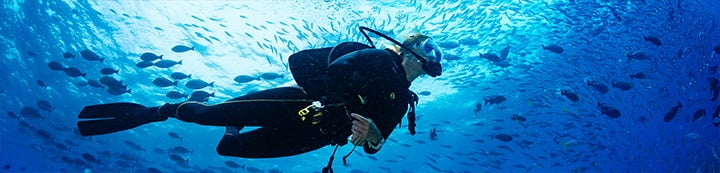1 - 10 of 10 Posts

#### Silly Car

·
##### Custom User Title
Joined
·
292 Posts
Discussion Starter · ·
I am in the process of trying to sort out a decanting excel spreadsheet and I would be grateful if someone would check my formula

Assuming

V1 = Donor cylinder size
P1 = Donor cylinder pressure
V2 = Recipient cylinder size
P2 = Current recipient cylinder pressure
P3 = Equalised pressure

Then

P3 = (V1 x P1) + (V2 x P2)
(V1 + V2)​

Therefore assuming 300bar 20 donor and 100b left in a twin 12 set, the equalised pressure would be 190.90 bar.

Also as 90.9 bar of gas has been shifted, then could I effectively calculate the re-cut mix if each cylinder had a different mix in it.

Although I would neve dive it without analysing, it would be great to be able to do this for planning purposes.

Cheers

#### 1693

·
##### Registered
Joined
·
11,575 Posts
Therefore assuming 300bar 20 donor and 100b left in a twin 12 set, the equalised pressure would be 190.90 bar
Correct
Also as 90.9 bar of gas has been shifted, then could I effectively calculate the re-cut mix if each cylinder had a different mix in it.
Yes. The new mix will be a blend of the original 100bar plus 90.9 bar of the mix from the donor cylinder
Although I would neve dive it without analysing, it would be great to be able to do this for planning purposes.
Very wise

#### Mal Bridgeman

·
##### A Moderate from 04/01/07-24/12/12
Joined
·
11,804 Posts
Looks about right to me.....you would get a smidgin more gas in the twinset if you filled it with the manifold closed....filled up one side....then filled up the other....

I would run the donor gas at the same percentage as in the twinset to remove any need to worry about the final result ....but would analyse anyway before putting the reg on it.

HTH
Mal

#### DannyB

·
##### Registered
Joined
·
1,790 Posts
I am in the process of trying to sort out a decanting excel spreadsheet and I would be grateful if someone would check my formula

Assuming

V1 = Donor cylinder size
P1 = Donor cylinder pressure
V2 = Recipient cylinder size
P2 = Current recipient cylinder pressure
P3 = Equalised pressure

Then

P3 = (V1 x P1) + (V2 x P2)
(V1 + V2)​
Therefore assuming 300bar 20 donor and 100b left in a twin 12 set, the equalised pressure would be 190.90 bar.

Also as 90.9 bar of gas has been shifted, then could I effectively calculate the re-cut mix if each cylinder had a different mix in it.

Although I would neve dive it without analysing, it would be great to be able to do this for planning purposes.

Cheers
That looks about right, but presuming that you have an isolator manifold on the twinset how can you get the pressure of the recpient cylinder higher than the donor and what is the equation?

Danny

#### 1693

·
##### Registered
Joined
·
11,575 Posts
If you're interested, I've knocked up a small spreadsheet that will calculate some 'What If' scenarios with using left over gas and air topping.

Ideal Gas Laws only, but I only use 232 Bar so it doesn't make too much difference.

Here's a screenshot. If you can see what's going on, you can use it; there's no documentationPM me with email addresses and I'll send a copy (it's 15k)
Usual caveats apply•Silly Car

#### Silly Car

·
##### Custom User Title
Joined
·
292 Posts
Discussion Starter · ·
That looks about right, but presuming that you have an isolator manifold on the twinset how can you get the pressure of the recpient cylinder higher than the donor and what is the equation?

Danny
You would need to use the same formula 3 times.

Once for decant one, manifold closed
Once for decant two, manifold closed, second cylinder
Finally for when you open the manifold

Eg Same scenario

P3i = (6000 + 1200)/ 32 = 225 bar
P3ii = ((225 * 20) + 1200) / 32 = 178 bar
P3iii = ((225 * 12) + (178 * 12)) / 24 = 201 bar

all ignoring decimal places, around 5% more (10bar) for taking your time#### DannyB

·
##### Registered
Joined
·
1,790 Posts
You would need to use the same formula 3 times.

Once for decant one, manifold closed
Once for decant two, manifold closed, second cylinder
Finally for when you open the manifold

Eg Same scenario

P3i = (6000 + 1200)/ 32 = 225 bar
P3ii = ((225 * 20) + 1200) / 32 = 178 bar
P3iii = ((225 * 12) + (178 * 12)) / 24 = 201 bar

all ignoring decimal places, around 5% more (10bar) for taking your timeI'm bored at work and came up with the following in excel....

B3=Donor Cylinder size
B4=Donor Cylinder Pressure
C3=Twinset cylinder size (of one of the cylinders ie twin 12's are 12)
C4=Twinset Pressure

Pressure of twinset at end of decant = ((C3*(((B3*(((C3*C4)+(B3*B4))/(B3+C3)))+(C3*C4))/(C3+B3)))+(C3*(((C3*C4)+(B3*B4))/(B3+C3))))/(C3*2)

Pressure left in donor cylinder = ((C3*C4)+((((C3*C4)+(B3*B4))/(B3+C3))*B3))/(C3+B3)

Danny

•Silly Car

#### Silly Car

·
##### Custom User Title
Joined
·
292 Posts
Discussion Starter · ·
I'm bored at work and came up with the following in excel....

B3=Donor Cylinder size
B4=Donor Cylinder Pressure
C3=Twinset cylinder size (of one of the cylinders ie twin 12's are 12)
C4=Twinset Pressure

Pressure of twinset at end of decant = ((C3*(((B3*(((C3*C4)+(B3*B4))/(B3+C3)))+(C3*C4))/(C3+B3)))+(C3*(((C3*C4)+(B3*B4))/(B3+C3))))/(C3*2)

Pressure left in donor cylinder = ((C3*C4)+((((C3*C4)+(B3*B4))/(B3+C3))*B3))/(C3+B3)

Danny
Crikey, I thought I was bored this afternoon!!!

Have a green#### Garf

·
##### GUE Instructor
Joined
·
9,260 Posts
I

Pressure of twinset at end of decant = ((C3*(((B3*(((C3*C4)+(B3*B4))/(B3+C3)))+(C3*C4))/(C3+B3)))+(C3*(((C3*C4)+(B3*B4))/(B3+C3))))/(C3*2)

Pressure left in donor cylinder = ((C3*C4)+((((C3*C4)+(B3*B4))/(B3+C3))*B3))/(C3+B3)

Danny
lol, good effort that man. you must be very bored indeed#### milldog

·
##### Utrinque Paratus
Joined
·
6,077 Posts
Sod that

•